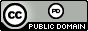시간 제한메모리 제한제출정답맞힌 사람정답 비율
2 초 512 MB167988260.741%

## 문제

Euler’s number (you may know it better as just $$e$$) has a special place in mathematics. You may have encountered $$e$$ in calculus or economics (for computing compound interest), or perhaps as the base of the natural logarithm, ln x, on your calculator.

While e can be calculated as a limit, there is a good approximation that can be made using discrete mathematics. The formula for $$e$$ is:

$e = \sum_{i=0}^{n}{\frac{1}{i!}} = \frac{1}{0!} + \frac{1}{1!} + \frac{1}{2!} + \frac{1}{3!} + \frac{1}{4!} + \cdots$

Note that 0! = 1. Now as n approaches ∞, the series converges to $$e$$. When n is any positive constant, the formula serves as an approximation of the actual value of $$e$$. (For example, at n = 10 the approximation is already accurate to 7 decimals.)

You will be given a single input, a value of n, and your job is to compute the approximation of e for that value of n.

## 입력

A single integer n, ranging from 0 to 10 000.

## 출력

A single real number – the approximation of e computed by the formula with the given n. All output must be accurate to an absolute or relative error of at most 10−12.

## 예제 입력 1

3


## 예제 출력 1

2.6666666666666665


## 예제 입력 2

15


## 예제 출력 2

2.718281828458995


## 출처• 문제의 오타를 찾은 사람: tpdnjs94
• 문제를 만든 사람: Joshua T. Guerin, Kathleen Ericson